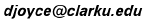# Continued Fractions

Continued fractions are a way of approximating real numbers by nearby rational numbers. The more terms there are in the continued fraction, the closer the rational number will be to the given real number.

Let x be the given real number. The first step in finding the continued fraction for x is to find the largest integer a less than or equal to x. Sometimes this is called the "greatest integer" in x and sometimes it's called the "floor" of x. We'll call it the floor. For example, the floor of 7/3 is 2. Note that the floor of an integer is that integer itself. For example, the floor of 3 is 3.

(If you can read these words, you're only seeing a captured image, not the real java applet! You need a browser that deals with java to move the points.)This figure utilizes the Geometry Applet.

April, 2006.
David E. Joyce
Department of Mathematics and Computer Science
Clark University
Worcester, MA 01610The address of this file is http://aleph0.clarku.edu/~djoyce/java/Geometry/contfract.html